HomeData EngineeringData DIYBeginners Tutorial On BERT

# Beginners Tutorial On BERT# Intent classification with LSTM

## Data

```labels = intent_data_label_train
plt.hist(labels)
plt.xlabel('intent')
plt.ylabel('nb samples')
plt.title('intent distribution')
plt.xticks(np.arange(len(np.unique(labels))));```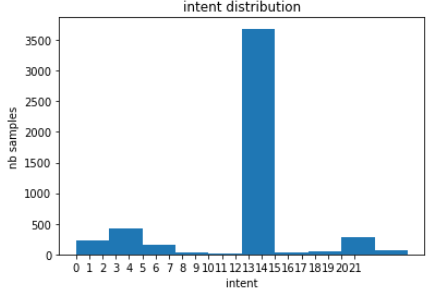## Multi-class classifier

```model_lstm = Sequential()
model_lstm.add(Embedding(vocab_in_size, embedding_dim, input_length=len_input_train))
model_lstm.add(LSTM(units))
model_lstm.add(Dense(nb_labels, activation='softmax'))
model_lstm.compile(loss='categorical_crossentropy', optimizer='adam', metrics=['accuracy'])
model_lstm.summary()

history_lstm = model_lstm.fit(input_data_train, intent_data_label_cat_train,
epochs=10,batch_size=BATCH_SIZE)```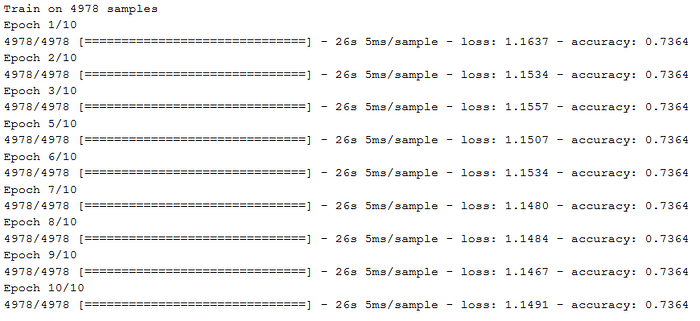```import scikitplot as skplt

def predict_intent(input_query, model=model_lstm, i2in=i2in_test, verbose=False):
sv = query_to_vector(input_query)
sv = sv.reshape(1,len(sv))
intent_idx = np.argmax(model.predict(sv), axis=1)
intent_label = i2in[intent_idx]
if verbose:
print(intent_label)
return intent_label, intent_idx

def evaluate_intent(queries, true_intents, model):
predicted_intents = []
for q in queries:
intent_label, intent_idx = predict_intent(q, model)
predicted_intents.append(intent_label)
skplt.metrics.plot_confusion_matrix(true_intents, predicted_intents, figsize=(12,15))

true_intents = [i2in_test[i] for i in intent_data_label_test]
evaluate_intent(query_data_test, true_intents, model_lstm)```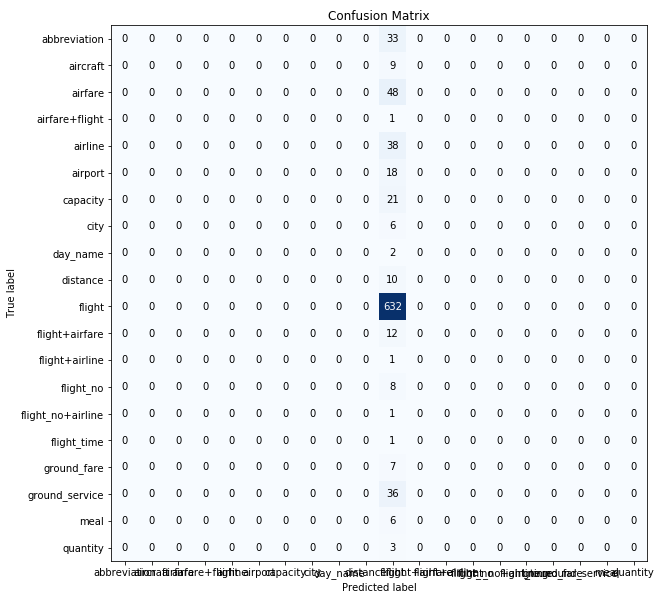## Binary classifier

```labels[labels==14] = -1
labels[labels!=-1] = 0
labels[labels==-1] = 1

plt.hist(labels)
plt.xlabel('intent')
plt.ylabel('nb samples')
plt.title('intent distribution after collapsing')
plt.xticks(np.arange(len(np.unique(labels))));```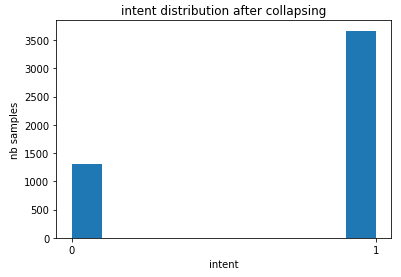```model_lstm2 = Sequential()
model_lstm2.add(Embedding(vocab_in_size, embedding_dim, input_length=len_input_train))
model_lstm2.add(LSTM(units))
model_lstm2.add(Dense(1, activation='sigmoid'))
model_lstm2.compile(loss='binary_crossentropy', optimizer='adam', metrics=['accuracy'])

history_lstm2 = model_lstm2.fit(input_data_train, labels, epochs=10, batch_size=BATCH_SIZE)```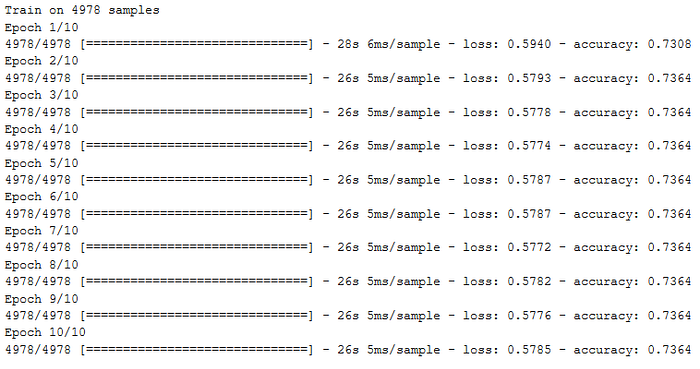```true_intents = ['flight' if i==14 else 'other' for i in intent_data_label_test]
predicted_intents = []
for q in query_data_test:
intent_label, intent_idx = predict_intent(q, model_lstm2)
predicted_intents.append('flight' if intent_idx==1 else 'other')

skplt.metrics.plot_confusion_matrix(true_intents, predicted_intents, figsize=(12,15))```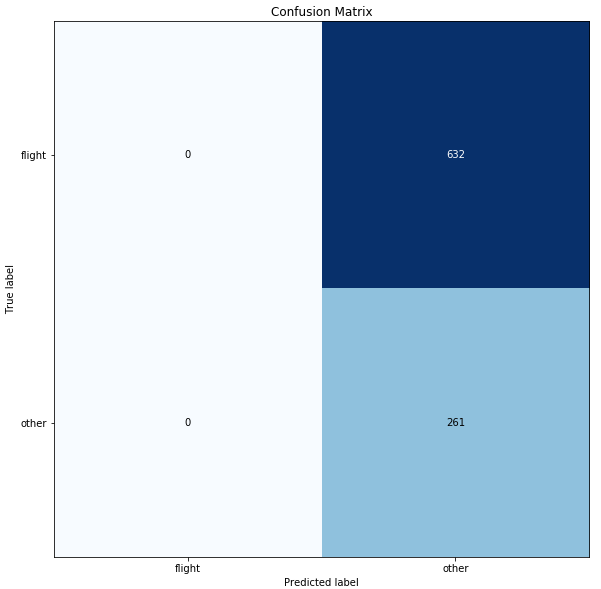# Intent Classification with BERT

## What is BERT?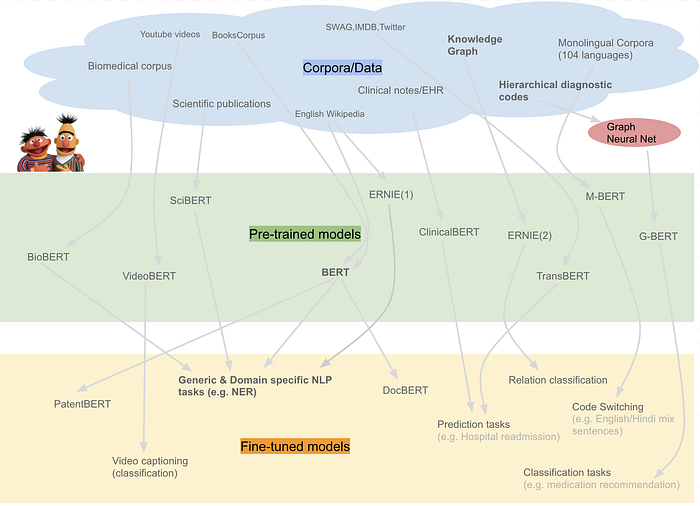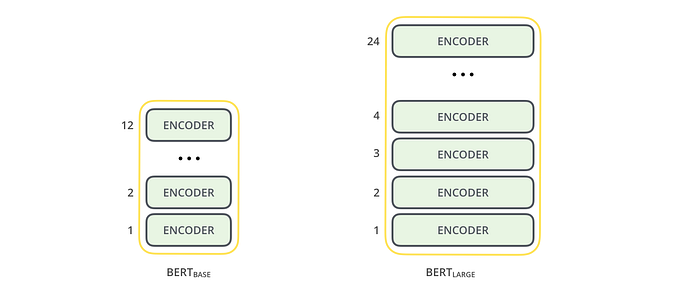## Preparing BERT environment

```# verify GPU availability
import tensorflow as tf

device_name = tf.test.gpu_device_name()
if device_name != '/device:GPU:0':
raise SystemError('GPU device not found')
print('Found GPU at: {}'.format(device_name))```
```# install
!pip install pytorch-pretrained-bert pytorch-nlp

# BERT imports
import torch
from torch.utils.data import TensorDataset, DataLoader, RandomSampler, SequentialSampler
from keras.preprocessing.sequence import pad_sequences
from sklearn.model_selection import train_test_split
from pytorch_pretrained_bert import BertTokenizer, BertConfig
from pytorch_pretrained_bert import BertAdam, BertForSequenceClassification
from tqdm import tqdm, trange
import pandas as pd
import io
import numpy as np
import matplotlib.pyplot as plt
% matplotlib inline

# specify GPU device
device = torch.device("cuda" if torch.cuda.is_available() else "cpu")
n_gpu = torch.cuda.device_count()
torch.cuda.get_device_name(0)```
```'[CLS]  i want to fly from boston at 838 am and arrive in denver at 1110 in the morning  [SEP]'

['[CLS]', 'i', 'want', 'to', 'fly', 'from', 'boston', 'at', '83', '##8', 'am', 'and', 'arrive', 'in', 'denver', 'at', '111', '##0', 'in', 'the', 'morning', '[SEP]']```
```# Set the maximum sequence length.
MAX_LEN = 128
# Pad our input tokens
input_ids = pad_sequences([tokenizer.convert_tokens_to_ids(txt) for txt in tokenized_texts],
maxlen=MAX_LEN, dtype="long", truncating="post", padding="post")
# Use the BERT tokenizer to convert the tokens to their index numbers in the BERT vocabulary
input_ids = [tokenizer.convert_tokens_to_ids(x) for x in tokenized_texts]
input_ids = pad_sequences(input_ids, maxlen=MAX_LEN, dtype="long", truncating="post", padding="post")```
```# Create attention masks
attention_masks = []
# Create a mask of 1s for each token followed by 0s for padding
for seq in input_ids:
seq_mask = [float(i>0) for i in seq]
attention_masks.append(seq_mask)```
```# Use train_test_split to split our data into train and validation sets for training
train_inputs, validation_inputs, train_labels, validation_labels = train_test_split(input_ids, labels,
random_state=2018, test_size=0.1)
train_masks, validation_masks, _, _ = train_test_split(attention_masks, input_ids,
random_state=2018, test_size=0.1)

# Convert all of our data into torch tensors, the required datatype for our model
train_inputs = torch.tensor(train_inputs)
validation_inputs = torch.tensor(validation_inputs)
train_labels = torch.tensor(train_labels)
validation_labels = torch.tensor(validation_labels)
train_masks = torch.tensor(train_masks)
validation_masks = torch.tensor(validation_masks)

# Select a batch size for training.
batch_size = 32

# Create an iterator of our data with torch DataLoader
train_data = TensorDataset(train_inputs, train_masks, train_labels)
train_sampler = RandomSampler(train_data)
train_dataloader = DataLoader(train_data, sampler=train_sampler, batch_size=batch_size)
validation_data = TensorDataset(validation_inputs, validation_masks, validation_labels)
validation_sampler = SequentialSampler(validation_data)
validation_dataloader = DataLoader(validation_data, sampler=validation_sampler, batch_size=batch_size)```
```# Load BertForSequenceClassification, the pretrained BERT model with a single linear classification layer on top.

model = BertForSequenceClassification.from_pretrained("bert-base-uncased", num_labels=nb_labels)
model.cuda()

# BERT model summary
BertForSequenceClassification(
(bert): BertModel(
(embeddings): BertEmbeddings(
(word_embeddings): Embedding(30522, 768, padding_idx=0)
(position_embeddings): Embedding(512, 768)
(token_type_embeddings): Embedding(2, 768)
(LayerNorm): BertLayerNorm()
(dropout): Dropout(p=0.1)
)
(encoder): BertEncoder(
(layer): ModuleList(
(0): BertLayer(
(attention): BertAttention(
(self): BertSelfAttention(
(query): Linear(in_features=768, out_features=768, bias=True)
(key): Linear(in_features=768, out_features=768, bias=True)
(value): Linear(in_features=768, out_features=768, bias=True)
(dropout): Dropout(p=0.1)
)
(output): BertSelfOutput(
(dense): Linear(in_features=768, out_features=768, bias=True)
(LayerNorm): BertLayerNorm()
(dropout): Dropout(p=0.1)
)
)
(intermediate): BertIntermediate(
(dense): Linear(in_features=768, out_features=3072, bias=True)
)
(output): BertOutput(
(dense): Linear(in_features=3072, out_features=768, bias=True)
(LayerNorm): BertLayerNorm()
(dropout): Dropout(p=0.1)
)
)
'
'
'
)
)
(pooler): BertPooler(
(dense): Linear(in_features=768, out_features=768, bias=True)
(activation): Tanh()
)
)
(dropout): Dropout(p=0.1)
(classifier): Linear(in_features=768, out_features=2, bias=True)
)```
```# BERT fine-tuning parameters
param_optimizer = list(model.named_parameters())
no_decay = ['bias', 'gamma', 'beta']
optimizer_grouped_parameters = [
{'params': [p for n, p in param_optimizer if not any(nd in n for nd in no_decay)],
'weight_decay_rate': 0.01},
{'params': [p for n, p in param_optimizer if any(nd in n for nd in no_decay)],
'weight_decay_rate': 0.0}
]

optimizer = BertAdam(optimizer_grouped_parameters,
lr=2e-5,
warmup=.1)

# Function to calculate the accuracy of our predictions vs labels
def flat_accuracy(preds, labels):
pred_flat = np.argmax(preds, axis=1).flatten()
labels_flat = labels.flatten()
return np.sum(pred_flat == labels_flat) / len(labels_flat)

# Store our loss and accuracy for plotting
train_loss_set = []
# Number of training epochs
epochs = 4

# BERT training loop
for _ in trange(epochs, desc="Epoch"):

## TRAINING

# Set our model to training mode
model.train()
# Tracking variables
tr_loss = 0
nb_tr_examples, nb_tr_steps = 0, 0
# Train the data for one epoch
for step, batch in enumerate(train_dataloader):
# Add batch to GPU
batch = tuple(t.to(device) for t in batch)
# Unpack the inputs from our dataloader
b_input_ids, b_input_mask, b_labels = batch
# Clear out the gradients (by default they accumulate)
optimizer.zero_grad()
# Forward pass
loss = model(b_input_ids, token_type_ids=None, attention_mask=b_input_mask, labels=b_labels)
train_loss_set.append(loss.item())
# Backward pass
loss.backward()
# Update parameters and take a step using the computed gradient
optimizer.step()
# Update tracking variables
tr_loss += loss.item()
nb_tr_examples += b_input_ids.size(0)
nb_tr_steps += 1
print("Train loss: {}".format(tr_loss/nb_tr_steps))

## VALIDATION

# Put model in evaluation mode
model.eval()
# Tracking variables
eval_loss, eval_accuracy = 0, 0
nb_eval_steps, nb_eval_examples = 0, 0
# Evaluate data for one epoch
for batch in validation_dataloader:
# Add batch to GPU
batch = tuple(t.to(device) for t in batch)
# Unpack the inputs from our dataloader
b_input_ids, b_input_mask, b_labels = batch
# Telling the model not to compute or store gradients, saving memory and speeding up validation
with torch.no_grad():
# Forward pass, calculate logit predictions
logits = model(b_input_ids, token_type_ids=None, attention_mask=b_input_mask)
# Move logits and labels to CPU
logits = logits.detach().cpu().numpy()
label_ids = b_labels.to('cpu').numpy()
tmp_eval_accuracy = flat_accuracy(logits, label_ids)
eval_accuracy += tmp_eval_accuracy
nb_eval_steps += 1
print("Validation Accuracy: {}".format(eval_accuracy/nb_eval_steps))

# plot training performance
plt.figure(figsize=(15,8))
plt.title("Training loss")
plt.xlabel("Batch")
plt.ylabel("Loss")
plt.plot(train_loss_set)
plt.show()```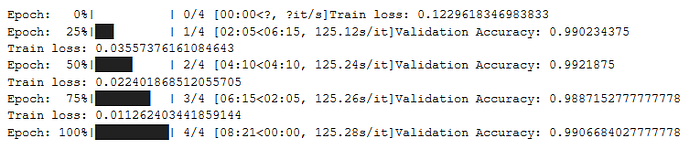BERT fine-tuned on the Intent Classification task for Natural Language Understanding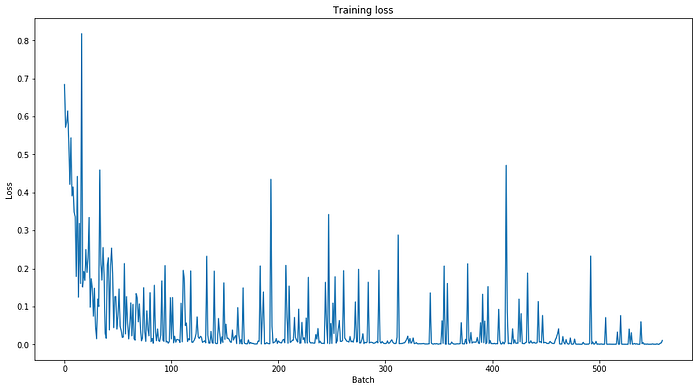```# load test data
sentences = ["[CLS] " + query + " [SEP]" for query in query_data_test]
labels = intent_data_label_test

# tokenize test data
tokenized_texts = [tokenizer.tokenize(sent) for sent in sentences]
MAX_LEN = 128
# Pad our input tokens
input_ids = pad_sequences([tokenizer.convert_tokens_to_ids(txt) for txt in tokenized_texts],
maxlen=MAX_LEN, dtype="long", truncating="post", padding="post")
# Use the BERT tokenizer to convert the tokens to their index numbers in the BERT vocabulary
input_ids = [tokenizer.convert_tokens_to_ids(x) for x in tokenized_texts]
input_ids = pad_sequences(input_ids, maxlen=MAX_LEN, dtype="long", truncating="post", padding="post")
# Create attention masks
attention_masks = []
# Create a mask of 1s for each token followed by 0s for padding
for seq in input_ids:
seq_mask = [float(i>0) for i in seq]
attention_masks.append(seq_mask)

# create test tensors
prediction_inputs = torch.tensor(input_ids)
prediction_masks = torch.tensor(attention_masks)
prediction_labels = torch.tensor(labels)
batch_size = 32
prediction_data = TensorDataset(prediction_inputs, prediction_masks, prediction_labels)
prediction_sampler = SequentialSampler(prediction_data)
prediction_dataloader = DataLoader(prediction_data, sampler=prediction_sampler, batch_size=batch_size)

## Prediction on test set
# Put model in evaluation mode
model.eval()
# Tracking variables
predictions , true_labels = [], []
# Predict
for batch in prediction_dataloader:
# Add batch to GPU
batch = tuple(t.to(device) for t in batch)
# Unpack the inputs from our dataloader
b_input_ids, b_input_mask, b_labels = batch
# Telling the model not to compute or store gradients, saving memory and speeding up prediction
with torch.no_grad():
# Forward pass, calculate logit predictions
logits = model(b_input_ids, token_type_ids=None, attention_mask=b_input_mask)
# Move logits and labels to CPU
logits = logits.detach().cpu().numpy()
label_ids = b_labels.to('cpu').numpy()
# Store predictions and true labels
predictions.append(logits)
true_labels.append(label_ids)

# Import and evaluate each test batch using Matthew's correlation coefficient
from sklearn.metrics import matthews_corrcoef
matthews_set = []
for i in range(len(true_labels)):
matthews = matthews_corrcoef(true_labels[i],
np.argmax(predictions[i], axis=1).flatten())
matthews_set.append(matthews)

# Flatten the predictions and true values for aggregate Matthew's evaluation on the whole dataset
flat_predictions = [item for sublist in predictions for item in sublist]
flat_predictions = np.argmax(flat_predictions, axis=1).flatten()
flat_true_labels = [item for sublist in true_labels for item in sublist]

print('Classification accuracy using BERT Fine Tuning: {0:0.2%}'.format(matthews_corrcoef(flat_true_labels, flat_predictions)))```

# Conclusion

This article has been published from the source link without modifications to the text. Only the headline has been changed.
- Advertisment -### Creating and Deploying an Ethereum Smart Contract

- Advertisment -